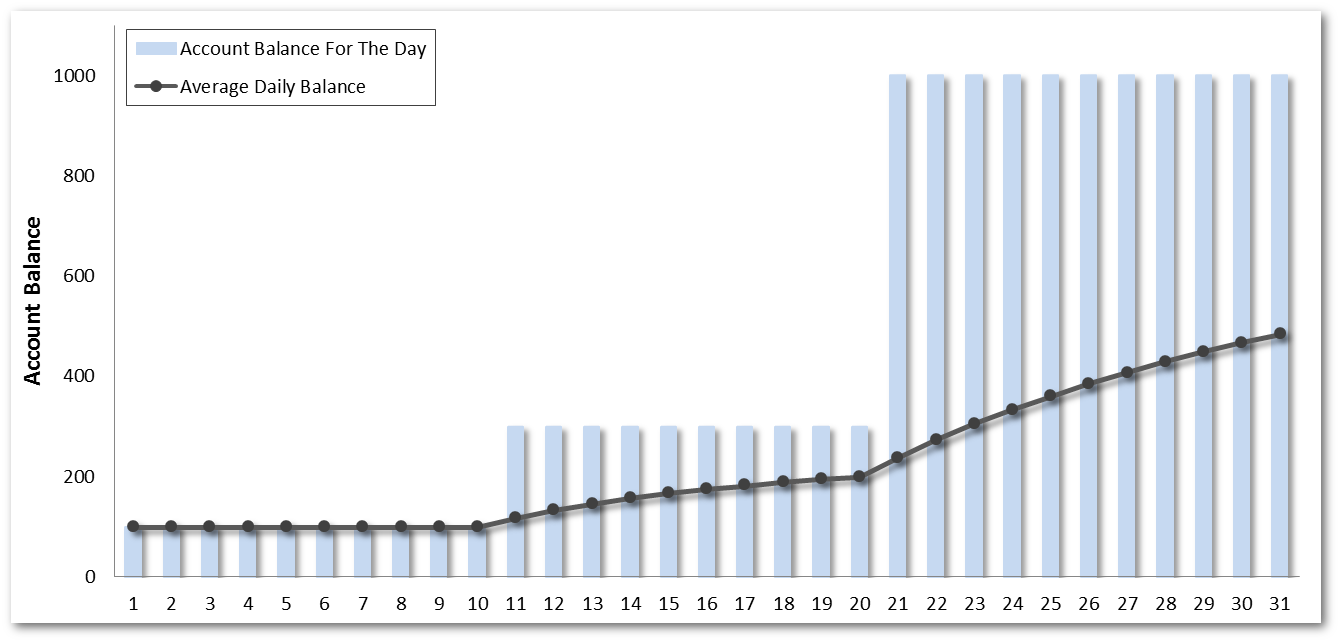﻿ Calculate Minimum Average Daily Balance (MADB) | POSB Singapore

# Calculate Minimum Average Daily Balance (MADB)

Minimum Average Daily Balance (MADB) is the minimum balance to be maintained in your account to avoid incurring Service Charge. Understand how it is calculated here.

Average Daily Balance is the total amount of daily balances in your account divided by the number of days in the month. To avoid incurring any service charges, a Minimum Average Daily Balance needs to be maintained in your account.

Below is a simple illustration on how Average Daily Balance is calculated:• Your account has a day-end balance of \$200 daily from 1 to 10 July, so total amount of daily balances for 10 days is:
\$200 x 10 Days = \$2,000.
• Your account has a day-end balance of \$300 daily from 11 to 20 July, so total amount of daily balances for 10 days is:
\$300 x 10 Days = \$3,000.
• Your account has a day-end balance of \$1,000 daily from 21 to 31 July, so total amount of daily balances for 11 days is:
\$1,000 x 11 Days = \$11,000
• Total amount of daily balances for 31 days in July is:
\$2,000 + \$3,000 + \$11,000 = \$16,000
• Average Daily Balance is therefore: \$16,000 divide by 31 (number of days in the month) = \$516.13
Check the required MADB for your account here and ensure that it is met to prevent incurring any Service Charge.